Published: 31 March 2017

# Research on ride comfort and safety of vehicle under limited conditions based on dynamical tire model

Yongjie Lu1
Wengang Zheng2
Enli Chen3
Junning Zhang4
1, 4Shijiazhuang Tiedao University, Shijiazhuang, P. R. China
2Tianjin Metro Company, Tianjin, P. R. China
3Key Laboratory of Traffic Safety and Control in Hebei, Shijiazhuang, P. R. China
Corresponding Authors:
Yongjie Lu, Wengang Zheng
Views 235

## 1. Introduction

Load transmission between vehicle and road is realized through tire/road contact patch. The hyperbolic structure of the tire and the large deformation characteristic of rubber material make the contact patch a dynamical boundary. During contact with the road, the tire goes through stretch, compression and bending to adapt top ups and downs of the road so as to generate complicated normal and tangential stress field on the contact interface. The stress distribution depends on wheel load, vehicle driving status, tire structure and tire/road friction status. Greenwood and Williamson (1966)  put forward the famous GW contact model and analyzed the contact mechanisms of irregular surfaces with an-isotropic characteristics, thus creating the research field of an-isotropic rough surface contact theories. Gal (2008)  described rough contact as micro area (5-100 μm) and macro area (300-1000 μm), established a rough road hysteresis friction factor model in consideration of characteristics of vertical acting force, conducted in-depth research on physical properties of hysteresis friction factor of rubber, and pointed out that the macro area was the main area influencing hysteresis friction factor and actual contact area. Carbone (2009)  proposed the improvement of GW model and developed the research of contact theory from micro area to macro area, thus laying a foundation for modeling through introduction of rubber friction to vehicle macro system. Heinrich (2008)  studied the mechanism concerning interaction between sliding tire and rough road, analyzed the influence of the energy loss from deformation of tire tread on the sliding friction factor and estimated the dynamic sliding friction factor with sliding area of the tire. Wei (2012)  applied Lagrange-Euler mixed description method to analyze speed field, accelerated speed field and contact deformation of tire big-deformation rolling contact structure, used orientation angle of the wheel as Cardan angle, relied on Lagrange description to obtain tire speed field containing rigid body rotation and elastic deformation, used Euler description to obtain deformation and stress of contact area, and completed dynamic analysis of rolling structure through information transmission of Euler network and Lagrange network. Xu (2013)  simplified tire/road actual contact area model and viscoelasticity energy loss, established an improved sliding friction factor model and intensively analyzed the action of tire and road characteristics on sliding friction factor. The interaction between tire and road is a very complicated dynamic process. During vehicle braking and turning, the friction status between tire and ground continuously changes. Adhesion friction force (adhesion area) and hysteresis friction force (sliding area) are nonlinear and changing dynamically. Therefore, it is difficult to carry out qualitative and quantitative description. To this end, good tire/road friction model cannot only reasonably express the dynamic behavior during tire/road contact motion but also be easily applied in vehicle control system. Tire/road friction model was classified as semi-empirical model and analytical model [7, 8]. Semi-empirical model can describe steady tire/road friction characteristics in a relatively accurate way since $\mu$-$s$ is usually obtained using curve fitting method based on experimental data. However, this model cannot reflect the influence of surface characteristics of road, tire pressure, hysteresis and other relevant factors on friction characteristics. It is actually a static friction model. Typical semi-empirical models include piecewise linearity model, Burckhardt model, Rill model, Magic model and Karnopp. Differential equation is usually adopted in analytical model to describe tire/road friction characteristics. It is actually a dynamical friction model. Typical analytical models include Armstrong model, Brush model, Dahl model, Bliaman-Sorine model and LuGre model. Abundant researches and application results indicated that LuGre model was the best among all analytical models. LuGre model boasted compact mathematical form and definite physical significance [9-11]. Deura (2004)  put forward a three-dimensional dynamical brush model based on LuGre friction model. This new model could simulate dynamical vertical and horizontal friction forces and alignment torques of tire in a relatively favorable way. Zuo Shuguang (2012)  established a tire-road finite element model based on LuGre dynamical friction model, conducted simulated analysis on lateral self-induced vibration of tire and research on influencing factors, and pointed out that lateral self-induced vibration would occur to the rolling tire under certain conditions so as to result in polygon abrasion of tire. The lumped LuGre tyre model and the vehicle kinematics are combined, the tyres internal deflection state is used to gain an accurate estimation (2016) . Yamashita (2015)  proposed the ANCF-LuGre tire model based on the absolute nodal coordinate formulation (ANCF),which is integrated with LuGre tire friction model, several numerical examples are presented to demonstrate the use of the in-plane ANCF-LuGre tire model for the longitudinal transient dynamics of tires under severe braking scenarios.Through combination of advantages and random steady-state characteristic (i.e. Stricbeck curve), LuGre model could accurately describe viscosity-sliding motion, friction hysteresis, pre-sliding displacement variable maximum static friction, steady oscillation ring, etc. as well as transient response of friction force of tire.

In this paper, a multi-body dynamical model of heavy vehicle was established. Actual vehicle data was adopted to carry out checking of virtual sample vehicle, dynamical friction characteristics of Stribeck were introduced to tire model during rolling contact between tire and road, and complete vehicle dynamic model truly reflecting dynamical contact between tire and road was put forward. Furthermore, correctness and availability of dynamical tire model was verified through comparison with classic Pac2002 tire model. Moreover, finite element method was applied to put forward a new 3D complicated road model construction method to construct roads of different classes and long-downhill paths of different S-curves. Simulated analysis of the influence of different speeds, different classes of random roads, different slopes and different adhesion road models on ride comfort of vehicle driving was implemented through utilization of event editor and drive control file, and speed limit standards under different conditions were put forward, so as to provide theoretical basis for design linearity and driving speed. Finally, the influence and changing rules of different speeds, different classes of random roads and different slopes on driving safety were discussed from the perspectives of each radial force of tire, alignment torque, sideslip angle and roll angle.

## 2. Establishment and checking of complete vehicle multi-body dynamic model

### 2.1. Modelling flow of multi-body vehicle model

Four types of files are needed during establishment of complete vehicle model in ADAMS/Car, namely, attribute file, template, subsystem and general assembly. Template is the foundation for establishment of each subsystem. Although the software is fitted with some demonstrative templates, it can far from satisfy customers’ demands. Therefore, it is necessary to construct templates and model. The modeling flow is shown in Fig. 1.

A private database shall be established before modeling to store template files established. The complete vehicle is divided into multiple different templates according to real vehicle structure of this heavy vehicle, including front suspension, tires, drive axle, plate spring, frame, steering system, rear suspension, driver’s cab, engine, etc. The multi-body dynamic model of complete vehicle generally assembled is shown in Fig. 2.

Fig. 1Flow chart of modeling of complete vehicle system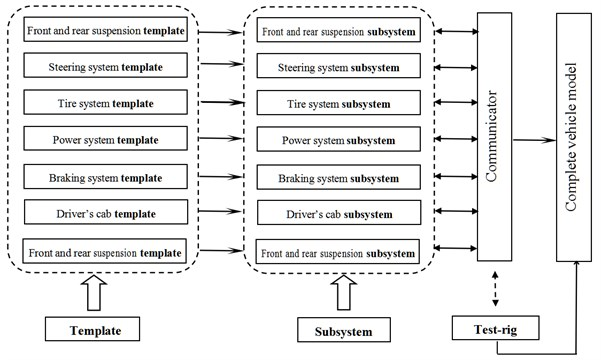Fig. 2Multi-body dynamic model of heavy vehicle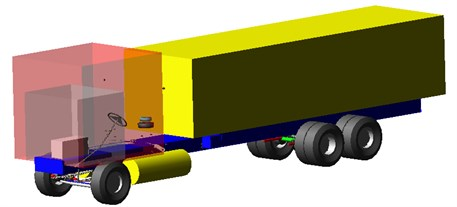### 2.2. Validating of complete vehicle model based on experimental data

The signal acquisition systems of vehicle test mainly include three-direction speed sensor, charge amplifier, signal acquisition instrument and computer. TSC-D3 speed sensor produced by Chengdu Zhongke Measurement and Control Co., Ltd. is adopted with frequency range of 1-500 Hz. Signal acquisition instrument is adopted to convert piezoelectric signal to digital signal. INV360DF acquisition, handling and analysis instrument produced by China Orient Institute of Noise & Vibration is adopted. The experiment site and acquisition instrument adopted in the experiment are shown in Figs. 3-6 represent site layout diagrams of speed sensor in measuring points of steering front axle head, balanced suspension middle axle head and rear axle head respectively.

Fig. 3Experiment and acquisition site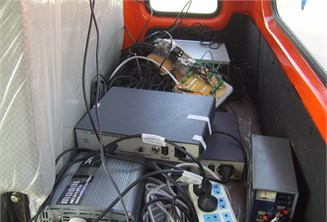Fig. 4Measuring point of front axle head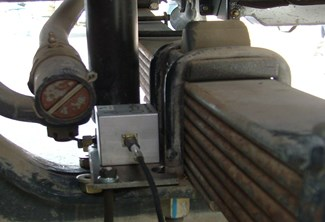Fig. 5Measuring point of middle axle head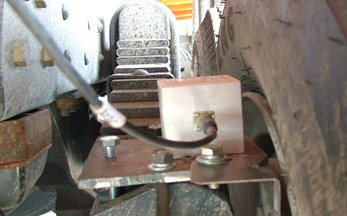Fig. 6Measuring point of rear axle head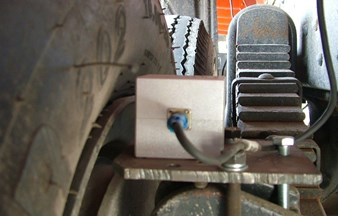The simulated graphs become close to experimental graphs through debugging of characteristic parameters of virtual sample vehicle including suspension rigidity, damping and pillowslip so as to check the accuracy of complete vehicle model established. Only a part of comparison results is selected here. Under the testing conditions of full load of vehicle, B-class random road and speed of 40 km/h, lateral and vertical velocity in time domain and frequency domain in each axle are compared with simulated data and the results are shown in Table 1.

Table 1Comparison between experiment and simulation

 Measuring position Maximum velocity (mm·s-1) Dominant frequency value (Hz) Simulation Experiment Simulation Experiment Lateral direction of left front axle 36.33 36.41 0.98 0.98 Vertical direction of left front axle 102.32 98.67 2.51 2.51 Lateral direction of left middle axle 41.58 42.37 0.987 1.241 Vertical direction of left middle axle 110.72 91.87 6.87 2.53 Lateral direction of left rear wheel 38.69 48.66 10.78 12.53 Vertical direction of left rear wheel 89.61 86.42 13.71 10.47

We can see from Table 1 that the experimental and simulated time domains and frequency domains have same trends under same velocity and road conditions, while the time domain and frequency value of simulated maximum velocity are very tiny. Take lateral direction of left front axle as an example. The simulated maximum speed is 36.33 mm/s. At this point, the frequency value is 0.98. The experiment results indicate that the maximum speed of vehicle at lateral direction of left front wheel is 36.41 mm/s and the frequency value is 0.98 at this point.

## 3. Construction of 3D S-curve and downhill road model

There are two methods establishing a 3D road in ADAMS/Car, namely, 3D equivalent volume method and 3D spline method. The principle of 3D equivalent volume road divides a road into a series of triangular planes for analysis as shown in Fig. 7. The 3D road is divided into 6 nodes and then four triangular elements A, B, C and D are therefore defined. The emphasis of equivalent volume method is placed on road node coordinates and number matrix. 3D coordinates and road friction conditions of the road are compiled through Road Builder. Fig. 8 indicates the spatial distribution of class-B 3D road.

A quick method applying finite element method and ADAMS environment to establish special sections was put forward in this paper. The simulated sections are described as “Introduced section (160 m)-S-shaped curve (1100 m)-Exit section (160 m)-Different downhill sections”. The curve radiuses S-shaped circles are 500 m and 528 m with azimuth angles of 43.855° and 45.626° respectively. The specific generation steps of 3D road system are shown as follows:

1) Draw road linearity in CAD and output it with format of .dxf as shown in Fig. 9;

2) Import .dxf file to ABAQUS to generate assembly body and divide grids as shown in Fig. 10;

3) Run Job to generate .inp file, draw three-direction coordinates of center line, import them to Excel and edit a model same as format of road point. Directly import to ADAMS. At this point, S-curve and downhill section road are already preliminarily formed as shown in Fig. 11;

4) Open Obstacle option in road editor and set up relevant parameters to obtain desired road surface shape and road roughness.

To this end, S-curve and downhill road are already constructed. At this point, event editor and drive control file can be edited to establish a 3D road system with different slopes and different road adhesion coefficient.

Fig. 73 D equivalent volume road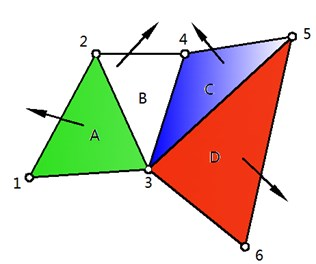Fig. 83 D Random Road (Class-B)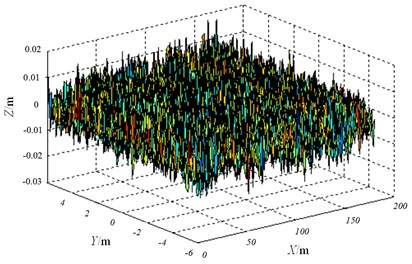Fig. 9CAD chart of curve and downhill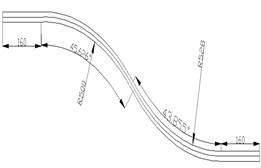Fig. 10Division grids of curve and downhill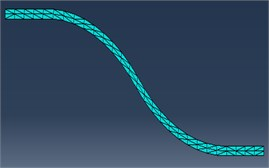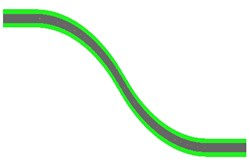## 4. Establishment of 2D LuGre dynamical tire model

The accurate description of characteristics of contact between tire and road has an important influence on vehicle driving performance (ride comfort and handling stability) and service performance and durability of road structure. Therefore, it is very important to establish a tire physical mechanic model “communicating” with the coupling between vehicle and road. The contact between vehicle tire and road is amidst a nonlinear and non-steady dynamical acting process. Therefore, it is very necessary to apply LuGre model to the vehicle modeling, which reflects the dynamical friction contact characteristics of transient variation between tire and road. LuGre dynamical friction model is modeled according to the idea of bristle model to better accurately describe steady and dynamical characteristics during contact between tire-ground friction. LuGre model is described using differential equation of first order, including Coulomb friction, viscous friction, pre-sliding, Stribeck effect (as shown in Fig. 12), variable static friction force, friction hysteresis and steady oscillation ring, rigidity coefficient of bristle; etc., where ${F}_{s}$ refers to maximum static friction, ${F}_{c}$ refers to coulomb friction, ${v}_{s}$ refers to Stribeck speed, ${v}_{r}$ refers to relative sliding speed between friction contact surfaces. Besides compact and concise expression, the friction model can better reflect the true friction phenomenon compared with before (as shown in Fig. 13), where ${F}_{z}$ refers to vertical load, $F$ refers to friction force, $\omega$ refers to tire rotation speed, $v$ refers to tire straight-line speed.

The friction force in LuGre tire dynamical friction model is represented as average elastic deformation of bristle, please refer to Eqs. (1-3):

1
$F={\delta }_{0}z+{\delta }_{1}\frac{dz}{dt}+{\delta }_{2}{v}_{r},$
2
$\frac{dz}{dt}=vr-\frac{{\delta }_{0}\left|{v}_{r}\right|}{g\left({v}_{r}\right)}z,$
3
$g\left({v}_{r}\right)={\mu }_{c}+\left({\mu }_{s}-{\mu }_{c}\right){e}^{-{\left|\frac{{v}_{r}}{{v}_{s}}\right|}^{{\alpha }_{s}}},$

where, ${\delta }_{0}$ refers to rigidity coefficient of bristle; ${\delta }_{1}$ refers to microcosmic damping coefficient of bristle; ${\delta }_{2}$ refers to relative viscous damping coefficient of bristle; $z$ refers to average elastic deformation of bristle; ${v}_{r}$ refers to relative sliding speed between friction contact surfaces; $g\left({v}_{r}\right)$ refers to forward sliding function; $F$ refers to friction; ${\mu }_{c}$ refers to coefficient of Coulomb friction; ${\mu }_{s}$ refers to static friction coefficient; ${v}_{s}$ refers to Stribeck speed which is ${v}_{s}=$5 m/s in the context; 1/3 refers to Stribeck index, indicating ${v}_{r}$ characteristic of steady friction. ${\alpha }_{s}\in$ [0.5, 2] under general circumstances and it is determined as ${\alpha }_{s}=$ 0.5 in the context.

Fig. 12Stribeck effect of LuGre tire friction force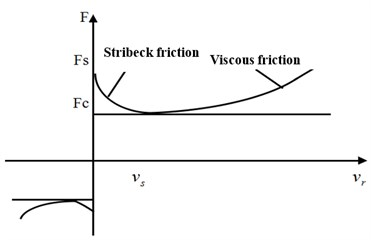Fig. 13Contact deformation of LuGre tire model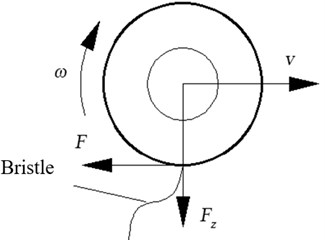Fig. 14Friction characteristic curve upon different frequencies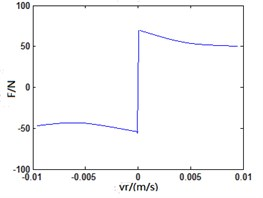a) 0.01 Hz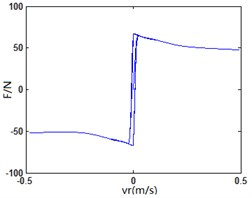b) 0.1 Hz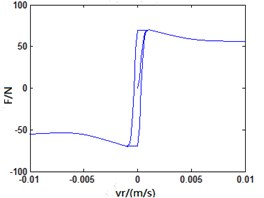c) 1 Hz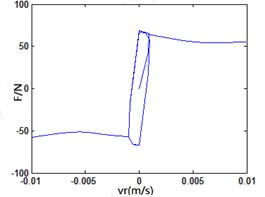d) 10 Hz

LuGre friction model is established in Matlab/Simulink. The sinusoidal variation of v under different frequencies is used as input to analyze the changing rules of friction force indicated in Fig. 12. When the frequency of $v$ is not big, LuGre friction model is similar to classic steady-state model; when the frequency of $v$ increases, the model indicates an obvious “hysteresis” phenomenon. Besides, the bigger the frequency and the more obvious “hysteresis” phenomenon will become. It indicates that LuGre friction model can well reflect transient response and “hysteresis” characteristic of friction between vehicle tire and road as shown in Fig. 14.

LuGre tire model is classified into four types, namely, lumped tire model, distribution tire model, an-isotropic tire model and steady-state model. When the tire is under joint condition, the unit module at moment $t$ moves along the tire rolling direction of axis $\xi$ and the bristle is deformed along both axis and axis $y$ under the condition that an-isotropic nature of tire is considered. It is recorded as ${z}_{x,y}\left(\zeta ,t\right)$. Then, the deformation of bristle of each unit module at moment $t+dt$ is ${z}_{x,y}\left(\zeta +d\zeta ,t+dt\right)$. See Fig. 15 for details, where $L$ is length of tire contact patch, $\alpha$ is sideslip angle. Therefore, $d{z}_{x,y}$ can be represented as Eq. (4):

4
$d{z}_{x,y}={z}_{x,y}\left(\zeta +d\zeta ,t+dt\right)-{z}_{x,y}\left(\zeta ,t\right).$

Fig. 15Graphs of LuGre tire with time change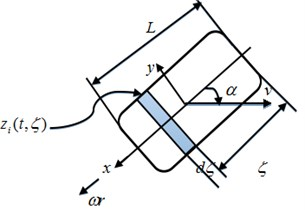a)$t$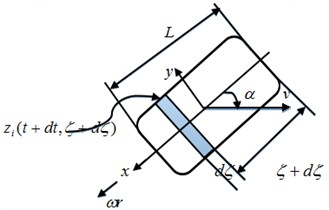b)$t+dt$

Represent $dz$ as function involving $\zeta$ and $x$:

5
$dz=\frac{\partial z}{\partial \zeta }d\zeta +\frac{\partial z}{\partial t}dt.$

Meanwhile:

6
$\frac{d\zeta }{dt}=\left|\omega r\right|.$

The following Eq. (7) can be obtained from Eq. (5) and Eq. (6):

7
$\frac{d{z}_{x,y}\left(\zeta ,t\right)}{dt}=\frac{\partial {z}_{x,y}\left(\zeta ,t\right)}{\partial t}+\frac{\partial {z}_{x,y}\left(\zeta ,t\right)}{\partial \zeta }\cdot \left|\omega r\right|.$

When Eq. (2) is combined, the following can be obtained:

8
$\begin{array}{c}\frac{\partial {z}_{x,y}\left(\zeta ,t\right)}{\partial t}={v}_{rx,y}\left(t\right)-\frac{{\delta }_{0}\left|{v}_{rx,y}\right|}{\theta \cdot {g}_{x,y}\left({v}_{rx,y}\right)}\cdot {z}_{x,y}\left(\zeta ,t\right)-\frac{\partial {z}_{x,y}\left(\zeta ,t\right)}{\partial \zeta }\cdot \left|\omega r\right|.\end{array}$

Where:

9
$\left\{\begin{array}{l}{v}_{rx}=\omega r-v\mathrm{c}\mathrm{o}\mathrm{s}\alpha ,\\ {v}_{ry}=-v\mathrm{s}\mathrm{i}\mathrm{n}\alpha ,\end{array}\right\$
10
${g}_{x,y}\left({v}_{rx,y}\right)=\left|\frac{{v}_{rx,y}}{v{}_{r}{}^{}}\right|\cdot g\left({v}_{r}\right).$

The expressions of longitudinal force ${F}_{x}$, lateral force ${F}_{y}$ and alignment torque ${M}_{z}$ of tire can be obtained as follows Eq. (11) and Eq. (12):

11
${F}_{x,y}={\int }_{0}^{L}{f}_{n}\left(\zeta \right)\left[{\delta }_{0x,y}{z}_{x,y}\left(\zeta ,t\right)+{\delta }_{1x,y}\frac{\partial {z}_{x,y}\left(\zeta ,t\right)}{\partial t}+{\delta }_{2x,y}{v}_{rx.y}\right]d\zeta ,$
12
${M}_{z}\left(t\right)={\int }_{0}^{L}{f}_{n}\left(\zeta \right)\left[{\delta }_{0y}{z}_{y}\left(\zeta ,t\right)+{\delta }_{1y}\frac{\partial {z}_{y}\left(\zeta ,t\right)}{\partial t}+{\delta }_{2y}{v}_{ry}\right]\cdot \left(\frac{L}{2}-\zeta \right)d\zeta ,$

where, ${f}_{n}\left(\zeta \right)$ refers to pressure density distribution function along the direction of contact patch of tire. When $v$ and $\omega$ are constant, the vehicle is under the stable driving status with $\partial z\left(\zeta ,t\right)/\partial t=0$ established. Then, the steady-state analytical solution of ${z}_{x,y}$ can be obtained:

13
${z}_{x,y}^{ss}\left(\zeta \right)={C}_{1x,y}\left(1-{e}^{-\frac{\zeta }{{C}_{2x,y}}}\right),$

where:

14
${C}_{1x,y}=\frac{{v}_{rx,y}\theta g\left({v}_{r}\right)}{\left|{v}_{r}\right|\cdot {\delta }_{0x,y}},$
15
${C}_{2x,y}=\left|\frac{\omega r}{{v}_{r}}\right|\cdot \frac{g\left({v}_{r}\right)}{{\delta }_{0x,y}}.$

The research indicated that the pressure distribution f tire under rolling status presented a “bell jar” shape. Aguilar-Martínez (2015)  provide an experimental setup for analysis of the tire-road contact area was designed and constructed. The experimental results confirmed the trapezoidal shape of the force distribution along the contact patch in the longitudinal direction.

This point has been studied by Deur (2002) , where calculations were made to find a trapezoidal distribution of force. The longitudinal force model and lateral force model of tire were basically free from the influence of heterogeneous distribution of pressure, while alignment force model was greatly influenced by pressure distribution. Therefore, uniform pressure distribution is obtained for longitudinal force model and lateral force model in this paper, while trapezoid distribution is obtained for vertical load in the alignment force expression, shown in Fig. 16, where ${f}_{n}\left(\xi \right)$ refers to the pressure distribution function along the direction of contact patch of tire, ${\xi }_{l}$refers to left inflection point of trapezoid pressure distribution, ${\xi }_{r}$ refers to right inflection point of trapezoid pressure distribution, ${f}_{n}{\left(\xi \right)}_{max}$ refers to the maximum value of the normal load distribution.

Fig. 16Diagram of trapezoid pressure distribution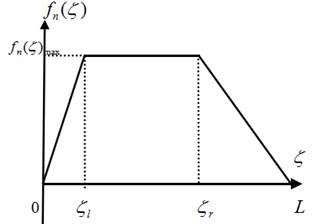The expression is shown as follows:

16
${f}_{n}\left(\zeta \right)=\left\{\begin{array}{ll}{\alpha }_{1}\zeta ,& 0\le \zeta \le {\zeta }_{l},\\ {f}_{n\mathrm{m}\mathrm{a}\mathrm{x}},& {\zeta }_{l}\le \zeta \le {\zeta }_{r},\\ {\alpha }_{2}\zeta +{\beta }_{2},& {\zeta }_{r}\le \zeta \le L.\end{array}\right\$

Accordingly, the expressions of longitudinal and lateral steady-state tire force and alignment torque are shown as follows:

17
${F}_{x,y}=\left\{{\delta }_{0x,y}{C}_{1x,y}\left[1-\frac{{C}_{2x,y}}{L}\left(1-{e}^{-\frac{L}{{C}_{2x,y}}}\right)\right]+{\delta }_{2x,y}{v}_{rx,y}\right\}{F}_{n},$
18
${M}_{z}=\left\{{\delta }_{0y}{C}_{1y}\frac{L}{2}\left[1-{C}_{3}+\frac{2L}{L+{\zeta }_{r}-{\zeta }_{l}}{C}_{4}^{2}\left(\frac{4{C}_{4}L-L}{\zeta l}-\frac{4{C}_{4}L+2{\zeta }_{l}-L}{{\zeta }_{l}}\cdot {e}^{-\zeta L\left({C}_{4}L\right)}\right)\right\right\$
$-\frac{4{C}_{4}L+2\zeta r-L}{L-\zeta r}\cdot {e}^{-\frac{\zeta r}{{C}_{4}L}}+\frac{4{C}_{4}L+L}{L-{\zeta }_{r}}{e}^{{}^{-\frac{1}{{C}_{4}}}}]+\frac{L}{2}\cdot \left(1-{C}_{3}\right){\delta }_{2y}{v}_{ry}}{F}_{n},$

where:

19
${C}_{3}=\frac{2\left({L}^{2}+{\zeta }_{r}L+{\zeta }_{r}-{{\zeta }_{l}}^{2}\right)}{3\left({L}^{2}+{\zeta }_{r}L-{\zeta }_{l}L\right)},$
20
${C}_{4}=\frac{\left|\omega r\right|{C}_{1y}}{L{v}_{ry}}.$

In LuGre tire model, ${F}_{n}$ and $L$ can be directly measured through experiment; ${v}_{r}$ can be calculated by formula according to parameters such as speed, wheel speed and slip angle of tire; ${v}_{s}$ and Stribeck index ${\alpha }_{s}$ were insignificantly influenced by experimental conditions. They are determined as constants in the context. Therefore, the parameters of model to identify are ${\mu }_{c}$, ${\mu }_{s}$, ${\delta }_{0}$, ${\delta }_{1}$ and ${\delta }_{2}$ where ${\mu }_{c}$, ${\mu }_{s}$ and ${\delta }_{2}$ are static parameters, while ${\delta }_{0}$ and ${\delta }_{1}$ are dynamic parameters. The identification of static parameters can be directly fitted using experimental data under steady-state condition, while it is relatively difficult to identify dynamic parameters. Currently, there is no very mature method yet.

### 4.1. Construction of LuGre dynamical tire model in Simulink

It is explained that it is very important to establish a tire physical mechanic model “communicating” with the coupling between vehicle and road. Therefore, it is very necessary to apply nonlinear LuGre model to the whole vehicle modeling, which reflects the dynamical friction contact characteristics of transient variation between tire and road. The tire model established in this paper includes two major parts, i.e. vehicle operation parameter subsystem and tire model parameter subsystem, involving 21 parameters in total. The vehicle operation parameter subsystem has 8 input parameters, namely, tire vertical load ${F}_{z}$, wheel speed $\omega$, wheel rolling radius $R$, relative speed ${v}_{r}$ and its components along direction $x$ and direction $y$, i.e. ${v}_{rx}$ and ${v}_{ry}$, tire contact patch length $L$ and road adhesion coefficient theta. LuGre tire model parameter subsystem has 13 input parameters, namely, longitudinal and lateral Coulomb friction coefficients ${\mu }_{cx}$ and ${\mu }_{cy}$, longitudinal and lateral static friction coefficients ${\mu }_{sx}$ and ${\mu }_{sy}$, Stribeck speed ${v}_{s}$, tire longitudinal and lateral rigidity coefficients ${\delta }_{0x}$ and ${\delta }_{0y}$, tire longitudinal and lateral damping coefficients ${\delta }_{1x}$ and ${\delta }_{1y}$, tire longitudinal and lateral relative viscous damping parameters ${\delta }_{2x}$ and ${\delta }_{2y}$ and trapezoid pressure distribution left and right inflection points ${\zeta }_{l}$ and ${\zeta }_{r}$. The foregoing is used as inputs and Eq. (17) and Eq. (18) are adopted to obtain the mathematical expressions of longitudinal force ${F}_{x}$, lateral force ${F}_{y}$ and alignment torque ${M}_{z}$. The calculation of each subsystem is based on their respective input parameters. MATLAB equation code is embedded in the built-in MATLAB function module in Simulink public module library to realize the calculation of relevant longitudinal force, lateral force and alignment torque as well as output the results. The specific logic diagram of dynamical tire model is shown in Fig. 17.

Fig. 17Construction of LuGre dynamical tire model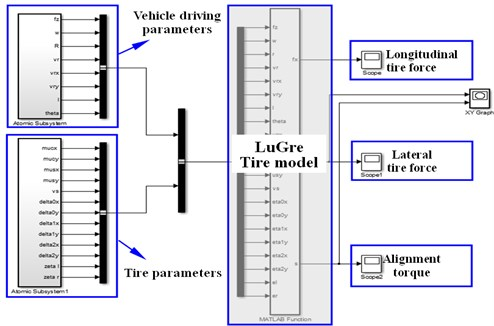### 4.2. Complete Vehicle dynamical model based on co-simulation

The core for realization of co-simulation interface between ADAMS/Car complete vehicle model and LuGre tire model lies in the selection of input and output parameters between the two. In the six-wheel LuGre tire model established in this paper, front and rear tire forces are marked as ${L}_{1}$, ${L}_{2}$, ${L}_{3}$, ${R}_{1}$, ${R}_{2}$ and ${R}_{3}$. The input parameters of ADAMS/Car model include longitudinal force ${F}_{{x}_{i}}$$\left(i\in {L}_{1},{L}_{2},{L}_{3},{R}_{1},{R}_{2},{R}_{3}\right)$, lateral force ${F}_{{y}_{i}}$$\left(i\in {L}_{1},{L}_{2},{L}_{3},{R}_{1},{R}_{2},{R}_{3}\right)$ and alignment torque ${M}_{{z}_{i}}$$\left(i\in {L}_{1},{L}_{2},{L}_{3},{R}_{1},{R}_{2},{R}_{3}\right)$ of six wheels, while the output parameters of ADAMS/Car model include vertical load ${F}_{{z}_{i}}$$\left(i\in {L}_{1},{L}_{2},{L}_{3},{R}_{1},{R}_{2},{R}_{3}\right)$ and slip angle. The principle of co-simulation between vehicle and dynamical tire model is shown in Fig. 18.

Fig. 18Co-simulation between vehicle and dynamical tire model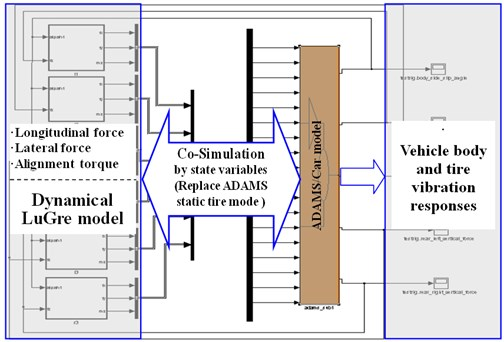### 4.3. Checking of Tire Model Based on Co-simulation

Simulated comparison of LuGre tire model and self-fitted tire complete vehicle model is carried out to compare with longitudinal force, lateral force, vertical force and alignment torque imposed on six wheels and further verify the correctness of combination of LuGre tire model to complete vehicle model. The simulated conditions are as follows: Speed as 60 km/h; S-curve and downhill; average slope as 2 %. Due to limitation of space, only comparison diagram of each index of left front wheel is provided herein as shown in Fig. 19.

It is thus clear that the trend and amplitude of three-direction tire force and alignment torque of LuGre type model are similar to those of self-fitted Pac2002 tire model, thus verifying availability of dynamic tire established in simulated analysis of dynamics of complete vehicle.

Fig. 19Comparison diagram of dynamical load of tire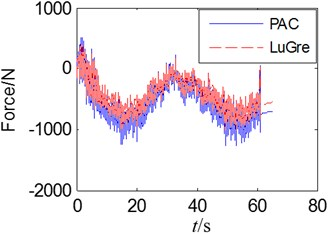a) Longitudinal force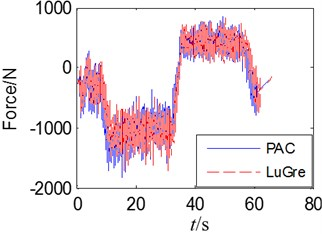b) Latern force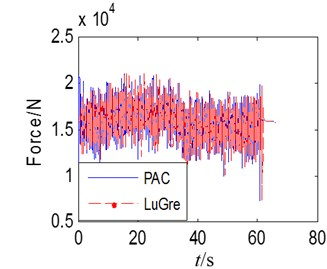c)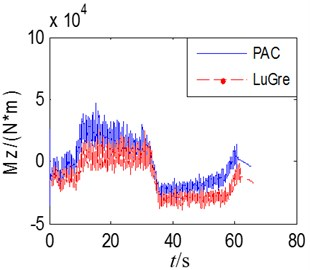d)

## 5. Analysis on ride comfort of vehicle in special sections

So far, the evaluation indexes and methods adopted at home and abroad on ride comfort of vehicle differ greatly especially for heavy vehicles. According to the stipulations of ISO2631-1/; 1997 (E), when the waveform peak factor of vibration signal is < 9, the root-mean-square value of weighted acceleration is adopted to evaluate the ride comfort of truck driving; when the waveform peak factor of vibration signal is > 9, the fourth root value is usually adopted to assist the evaluation of ride comfort. The relationships of root-mean-square value of weighted acceleration and weighted vibration level ${L}_{eq}$ with people’s subjective feelings have been provided in relevant international standard.

### 5.1. Speed simulation for ride comfort and result analysis

On the most common class-B and class-C random asphalt roads, the vehicle is driven on slope-free road of S-curve built at different speeds to analyze influence of different speeds on ride comfort of vehicle. The analysis results are shown in Figs. 20-23.

The following conclusions are drawn through analysis:

1) We can see from Fig. 20 and Fig. 21 that the vehicle driving speed has a relatively significant influence on ride comfort. With the increase of speed, the three-direction acceleration of driver’s cab presents a rising trend. The influence on lateral force is the most sensitive during high-speed stage; with the increase of speed, vehicle’s ride comfort becomes worse. On class-B road, when the speeds are 20 km/h, 40 km/h and 60 km/h, ${a}_{w}<$0.315 and ${L}_{aw}<$110 dB. At this point, the driver does not feel uncomfortable. When the speed reaches 80 km/h, ${a}_{w}=$0.629 and ${L}_{aw}=$115.838. At this point, the driver begins to feel uncomfortable. When the speed reaches 100 km/h, ${a}_{w}=$1.345 and ${L}_{aw}=$122.576. At this point, the driver feels very uncomfortable. On class-C road, when the speed is 20 km/h, ${a}_{w}=$0.805 and ${L}_{aw}=$118.123. The driver feels uncomfortable at this speed. When the speed is 100 km/h, ${a}_{w}=$ 2.224 and ${L}_{aw}=$126.931. At this point, the driver feels extremely uncomfortable.

2) We can see from Fig. 22 and Fig. 23 that the road has an extremely big influence on ride comfort. When the speed is less than 60 km/h, the change is not significant. When the speed is greater than 60 km/h, the total weighted acceleration rises perpendicularly. The total weighted root mean square of acceleration at same speed differs greatly as well. The poorer the road and the bigger the weighted root mean square value of acceleration, the bigger the weighted vibration level and the poorer the ride comfort will become. It is thus clear that lowering of speed and improving of roughness of road surface are effective approaches to improve ride comfort. In order to improve driver’s comfort, the speed of full-load goods van shall be best controlled within 60 km/h on class-B road. On a class-C road, the driver feels uncomfortable even if the speed is only 20 km/h. Therefore, road roughness has a great influence on ride comfort of the vehicle.

Fig. 20Three-direction acceleration of driver’s cab (Class-B Road)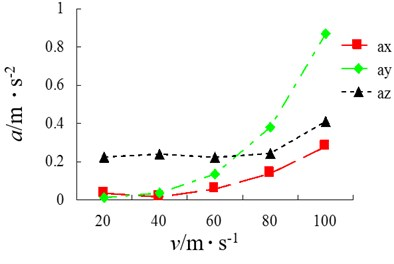Fig. 21Three-direction acceleration of driver’s cab (Class-C Road)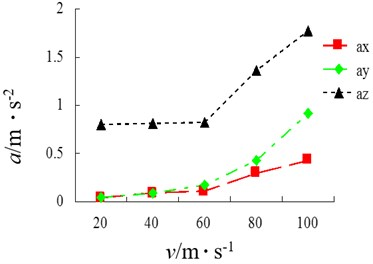Fig. 22Total weighted acceleration of driver’s cab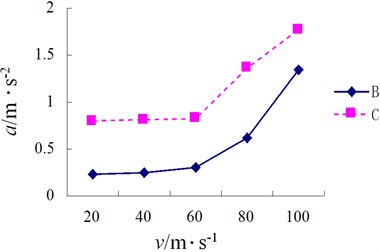Fig. 23Total weighed vibration level of driver’s cab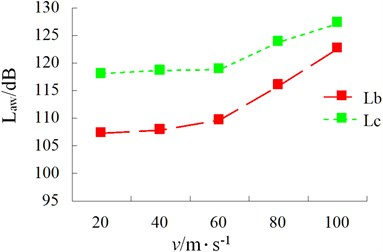### 5.2. Influence of different slopes on ride comfort of vehicle

As for roads with slope of 2 %-10 %, simulation of different slopes is conducted to obtain each acceleration and power spectral density of driver’s cab. The ride comfort of driver under different slopes and at each speed is shown in Table 2. In order to further analyze the reason of instability of vehicles in special sections, the different braking forces of the vehicle at speed of 40 km/h are analyzed as shown in Fig. 24.

Table 2Weighted root-mean-square value of driver’s cab. (Note: √ indicates no discomfort, while × indicates failed simulation)

 Slope / % 20 (km/h) 40 (km/h) 60 (km/h) 80 (km/h) 100 (km/h) 2 √ √ 0.29 0.62 1.61 3 √ √ 0.26 0.63 1.74 4 √ √ 0.26 0.65 × 5 √ √ 0.31 × × 6 √ √ 0.41 × × 7 √ 0.23 × × × 8 √ 0.24 × × × 9 0.21 0.27 × × × 10 × × × × ×

Fig. 24Braking force under different slopes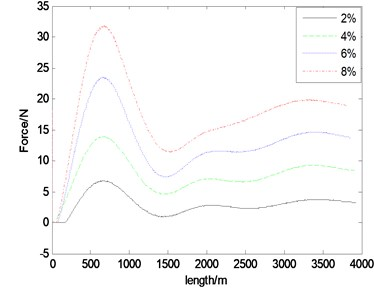The following conclusions are drawn through comparison with above mentioned analysis:

(1) When the slope is 2 % and the speed is lower than 60 km/h, the driver does not feel uncomfortable. When the speed is 70 km/h, ${a}_{w}=$0.38 m/s2. At this point, the driver feels a little uncomfortable. When the speed is 80 km/h, ${a}_{w}=$ 0.62 m/s2. At this point, the driver is very uncomfortable. When the speed is 100 km/h, ${a}_{w}=$1.60 m/s2. At this point, the driver is extremely uncomfortable. Therefore, in consideration of ride comfort of driver, the speed shall be restricted as lower than 70 km/h.

(2) When the slope is 4 % and the speed is lower than 60 km/h, the driver does not feel uncomfortable. When the speed is 70 km/h, ${a}_{w}=$0.443 m/s2. At this point, the driver feels a little uncomfortable. When the speed is 80 km/h, ${a}_{w}=$0.65 m/s2. At this point, the driver is very uncomfortable. When the speed is 90 km/h, ${a}_{w}=$2.35 m/s2. At this point, the driver is extremely uncomfortable. When the speed is 100 km/h, simulation fails. Therefore, in consideration of ride comfort of driver, the speed shall be restricted as lower than 60 km/h and it is strictly prohibited to exceed 90 km/h.

(3) When the slope is 6 % and the speed is lower than 60 km/h, the driver does not feel uncomfortable. When the speed is 60 km/h, ${a}_{w}=$0.413 m/s2. At this point, the driver feels a little uncomfortable. When the speed is 70 km/h, the brake fails to result in failure of simulation due to excessive braking force. Therefore, in consideration of ride comfort of driver, the speed shall be restricted as lower than 50 km/h and it is strictly prohibited to exceed 70 km/h.

(4) When the slope is 8 % and the speed is lower than 50 km/h, the driver does not feel uncomfortable. When the speed is 60 km/h, the brake fails to result in failure of simulation due to excessive braking force. Therefore, in consideration of ride comfort of driver, the speed shall be restricted as lower than 50 km/h and it is strictly prohibited to exceed 50 km/h.

(5) When the slope is 10 % and the speeds are 20 km/h, 30 km/h and 40 km/h, the brake fails to result in failure of simulation due to excessive braking force. Therefore, during road design, the slope shall not be too big.

It is thus clear that slope not only influences ride comfort but also causes very serious consequences if it is excessive to result in failure of braking. Therefore, big-slope road shall be avoided as much as possible. Stipulations concerning maximum road slope and speed are shown in Table 3 after consulting of stipulations set out in Detailed Rules for Design of Road Project Line (JTG-TD20-2006)  or Technical Standard for Road Engineering (JTG B01-2014) . The simulation results reflect the relationship between maximum slope and speed in Table 4. The correctness of model and simulation results is thus further explained.

 Design speed / km·h-1 120 100 80 60 40 30 20 Max slope / % 3 4 5 6 7 8 9

Table 4Maximum road slope calculated based on dynamic tire

 Design speed / km·h-1 100 80 60 40 20 Slope / % 3 4 6 7 9

## 6. Simulation of driving safety in special sections

### 6.1. Influence of different speeds on driving safety

Simulation condition: S-curve, class-B, dry asphalt road. The results of each index of simulation of influence of speed on vehicle driving safety are shown in Figs. 25-30.

Speed has a relatively obvious influence on lateral force of each tire of vehicle. With the increase of speed, the sudden changes of tire force, alignment torque, sideslip angle and roll angle become increasingly obvious in S-curve and the tire bears increasing impact force.

Fig. 25RMS of Wheel horizontal force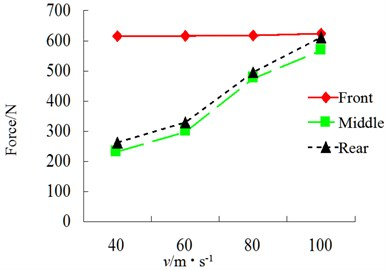Fig. 26RMS of wheel lateral force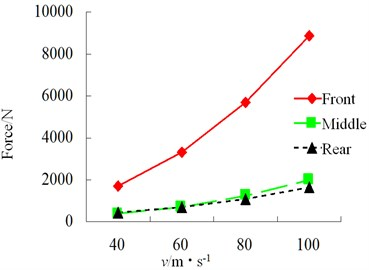Fig. 27RMS of wheel vertical force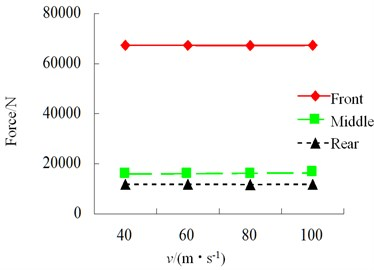Fig. 28RMS of wheel alignment torque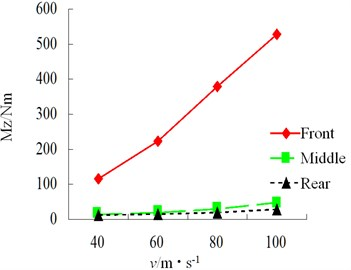Fig. 29RMS of wheel sideslip angle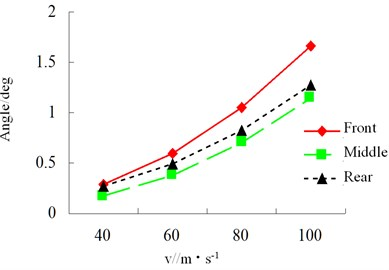Fig. 30RMS of wheel roll angle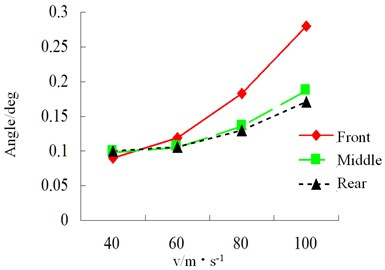Fig. 31RMS of wheel horizontal force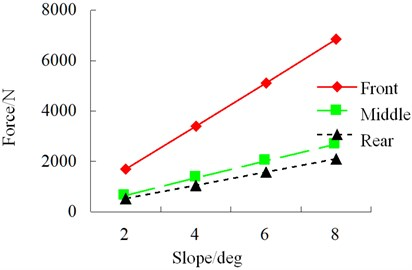Fig. 32RMS of wheel lateral force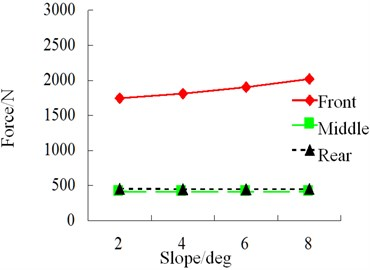Fig. 33RMS of wheel vertical force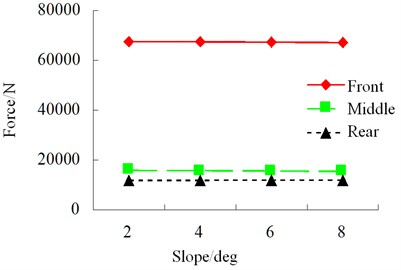Fig. 34RMS of wheel alignment torque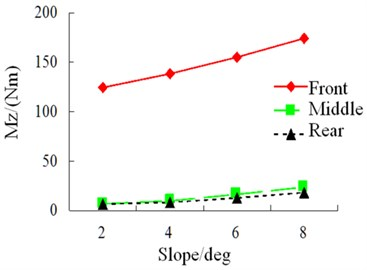Fig. 35RMS of wheel sideslip angle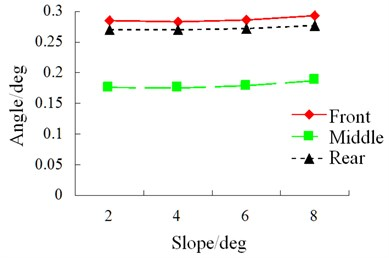Fig. 36RMS of wheel roll angle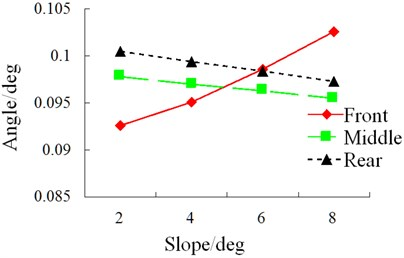### 6.2. Influence of slope on driving safety

Simulation condition: S-curve, class-B random road, speed 40 km/h. The results of each index of simulation of influence of slope on vehicle driving safety are shown in Figs. 31-36.

During driving, the road slope has a relatively obvious influence on horizontal force and roll angle of the vehicle. However, in an S-curve, sudden changes still occur to lateral force, alignment torque and sideslip angle. Therefore, road scope has a great influence on vehicle safety.

## 7. Conclusions

1) Influence of different speeds on ride comfort. When the vehicle is full-load and driven on a random class-B road involving long-downhill slope of S-curve, the speed shall be lower than 60 km/h. When the vehicle is driven on a class-C road, the driver feels uncomfortable even if the speed is only 20 km/h. Therefore, the curve road has a relatively significant influence on ride comfort of the vehicle.

2) Influence of different slopes on ride comfort. Road slope not only influences ride comfort but also causes very serious consequences if it is excessive to result in failure of braking. Therefore, big-slope road shall be avoided as much as possible.

3) Influence of different speeds and slopes on vehicle safety. With changes of vehicle driving speed, road class and change of slope, each index changes significantly especially during the S-curve where each index changes suddenly.

The transient responses of longitudinal sliding and lateral sliding of vehicle under complicated line conditions are simulated in this paper. In the next step, joint control objectives including vehicle’s active safety, handling stability and ride comfort can be realized through formulation of vehicle active steering, wheel sliding rate and semi-active suspension control strategies.

#### Cited by

Influence of vehicle-road coupled vibration on tire adhesion based on nonlinear foundation
Truck Handling Stability Simulation and Comparison of Taper-Leaf and Multi-Leaf Spring Suspensions with the Same Vertical Stiffness

24 July 2016
Accepted
29 September 2016
Published
31 March 2017
SUBJECTS
Vibration in transportation engineering
Keywords
heavy vehicle
limited conditions
dynamical friction
LuGre tire
Acknowledgements

This work was supported by National Natural Science Foundation of China (Grant Nos. 11572207, 11472180, 11302138) and Natural Science Foundation of Hebei Province (Grant No. A2016210103).